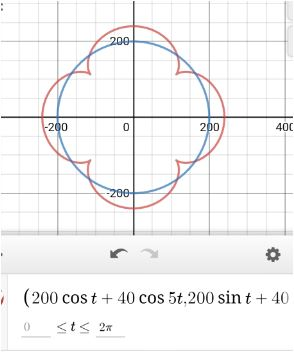# Many carnival have a version of the double Ferris wheel . A large central arm rotates clockwise ....

## Question:

Many carnival have a version of the double Ferris wheel . A large central arm rotates clockwise . At the end of the central wheel arm is a ferris wheel that rotates clockwise around the arm. assume that the central arm ahas length {eq}200 {/eq} feet and rotates about its center.assume that the wheels have radius {eq}40 {/eq} feet and rotate at the same speed as the central arm . Find the parametric equation for the position of a rider and graph the rider path.

## Finding Parametric Equation:

This problem involves finding the parametric equation of a given scenario. The idea is to identify the motion of the rider in a double Ferris wheel, write the parametric equation of the rider and then graph it using a plotter.

Given a double Ferris wheel, where a small wheel of 40 feet radius is attached to the end of a large arm of a wheel with a radius of 200 feet. Given, they rotate at the same speed.

Hence the ratio of angular velocities are -{eq}\displaystyle \omega_1 R_1 = \omega_2 R_2 \Rightarrow \omega_2 = \frac{ \omega_1 200}{40} = 5 \omega_1 {/eq}

Let's say the angular velocity of the large arm is 1, so the angular velocity of the smaller wheel is 5.

Thus, the parametric equation of the center of the second wheel ( which is attached at the end of the larger arm ) is

{eq}\displaystyle (x,y) = ( 200 \cos t, 200 \sin t) {/eq}

Now, the rider is actually riding on the 40 feet wheel, so we have the parametric equation of rider to be -

{eq}\displaystyle (x,y) = ( 200 \cos t + 40 \cos 5t, 200 \sin t + 40 \sin 5t) {/eq}

The graph of the rider is -The red curve is the graph of the rider, the blue curve is the giant wheel.